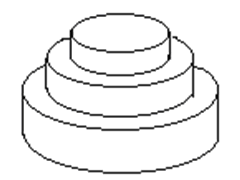# #1019. 「一本通 1.3 例 2」生日蛋糕

### 题目描述

Mr.W 要制作一个体积为 $N\pi$ 的 $M$ 层生日蛋糕，每层都是一个圆柱体。 设从下往上数第 $i$ 蛋糕是半径为 $R_i$，高度为 $H_i$ 的圆柱。当 $i\u003CM$ 时，要求 $R_i>R_{i+1}$且 $H_i >H_{i+1}$。由于要在蛋糕上抹奶油，为尽可能节约经费，我们希望蛋糕外表面（最下一层的下底面除外）的面积 $Q$ 最小。 令 $Q =Sπ$ ，请编程对给出的 $N$ 和 $M$ ，找出蛋糕的制作方案（适当的 $R_i$ 和 $H_i$ 的值），使 $S$ 最小。（除 $Q$ 外，以上所有数据皆为正整数）### 样例

#### 样例输入

100
2

#### 样例输出

68